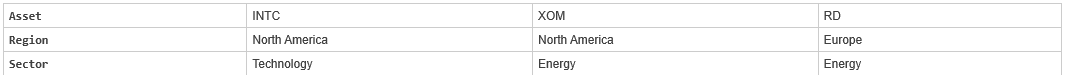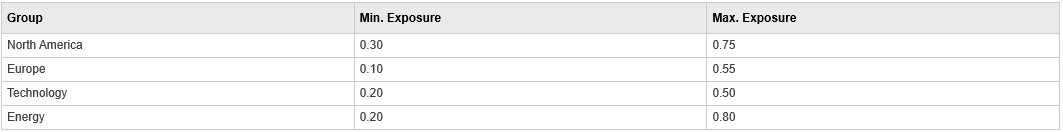# pcgcomp

Linear inequalities for asset group comparison constraints

## Syntax

``[A,b] = pcgcomp(GroupA,AtoBmin,AtoBmax,GroupB)``

## Description

As an alternative to `pcgcomp`, use the Portfolio object (`Portfolio`) for mean-variance portfolio optimization. This object supports gross or net portfolio returns as the return proxy, the variance of portfolio returns as the risk proxy, and a portfolio set that is any combination of the specified constraints to form a portfolio set. For information on the workflow when using Portfolio objects, see Portfolio Object Workflow.

example

````[A,b] = pcgcomp(GroupA,AtoBmin,AtoBmax,GroupB)` specifies that the ratio of allocations in one group to allocations in another group is at least `AtoBmin` to `1` and at most `AtoBmax` to `1`. Comparisons can be made between an arbitrary number of group pairs `NGROUPS` comprising subsets of `NASSETS` available investments.If `pcgcomp` is called with fewer than two output arguments, the function returns `A` concatenated with `b` `[A,b]`.```

## Examples

collapse all

Use the following assets and groupings.Make the North American energy sector compose exactly 20% of the North American investment.

```% INTC XOM RD GroupA = [ 0 1 0 ]; % North American Energy GroupB = [ 1 1 0 ]; % North America AtoBmin = 0.20; AtoBmax = 0.20; [A,b] = pcgcomp(GroupA, AtoBmin, AtoBmax, GroupB)```
```A = 2×3 0.2000 -0.8000 0 -0.2000 0.8000 0 ```
```b = 2×1 0 0 ```

Portfolio weights of 40% for INTC, 10% for XOM, and 50% for RD satisfy the constraints.

## Input Arguments

collapse all

Grouping A, specified as a number of groups (`NGROUPS`) by number of assets (`NASSETS`) vector of groups to compare. Each row specifies a group. For a specific group, `Group(i,j) = 1` if the group contains asset `j`; otherwise, `Group(i,j) = 0`.

Data Types: `double`

Minimum ratios, specified as a scalar or `NGROUPS`-long vectors of minimum ratios of allocations in `GroupA` to allocations in `GroupB`. `NaN` indicates no constraint between the two groups. Scalar bounds are applied to all group pairs. The total number of assets allocated to `GroupA` divided by the total number of assets allocated to `GroupB` is >= `AtoBmin` and <= `AtoBmax`.

Data Types: `double`

Maximum ratios, specified as a scalar or `NGROUPS`-long vectors of maximum ratios of allocations in `GroupA` to allocations in `GroupB`. `NaN` indicates no constraint between the two groups. Scalar bounds are applied to all group pairs. The total number of assets allocated to `GroupA` divided by the total number of assets allocated to `GroupB` is >= `AtoBmin` and <= `AtoBmax`.

Data Types: `double`

Grouping B, specified as a number of groups (`NGROUPS`) by number of assets (`NASSETS`) vector of groups to compare. Each row specifies a group. For a specific group, `Group(i,j) = 1` if the group contains asset `j`; otherwise, `Group(i,j) = 0`.

Data Types: `double`

## Output Arguments

collapse all

Lower bound, returned as a matrix such that ```A*PortWts' <= b```, where `PortWts` is a `1`-by-`NASSETS` vector of asset allocations.

Upper bound, returned as a vector such that ```A*PortWts' <= b```, where `PortWts` is a `1`-by-`NASSETS` vector of asset allocations.

Introduced before R2006a